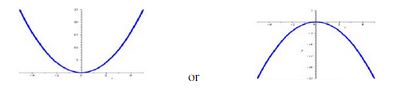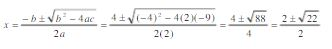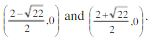Ontario Tech acknowledges the lands and people of the Mississaugas of Scugog Island First Nation.

We are thankful to be welcome on these lands in friendship. The lands we are situated on are covered by the Williams Treaties and are the traditional territory of the Mississaugas, a branch of the greater Anishinaabeg Nation, including Algonquin, Ojibway, Odawa and Pottawatomi. These lands remain home to many Indigenous nations and peoples.

We acknowledge this land out of respect for the Indigenous nations who have cared for Turtle Island, also called North America, from before the arrival of settler peoples until this day. Most importantly, we acknowledge that the history of these lands has been tainted by poor treatment and a lack of friendship with the First Nations who call them home.

This history is something we are all affected by because we are all treaty people in Canada. We all have a shared history to reflect on, and each of us is affected by this history in different ways. Our past defines our present, but if we move forward as friends and allies, then it does not have to define our future.

# Parabolas

A parabola is a conic section. The following two plots are examples of parabolas:Parabolas have the property that when transformed (stretched/compressed) along a direction parallel or perpendicular to its axis, the curve remains a parabola. For example, a line also has this property, but a circle does not. If a line is stretched or compressed, it retains its shape; a circle does not.

Any function of the form f(x) = a(x - h)2 + k is in the square family because it is a transformation of f(x) = x2. The graph of any function in the square family is called a parabola. Therefore, the graph of any quadratic function is a parabola.

## Opening and Vertex of a Parabola

There are two forms of the quadratic function, where one form is f(x) = a(x - h)2 + k and the other form is f(x) = ax2 + bx + c. If a > 0, the graph of f(x) = a(x - h)2 + k opens upward; if a < 0, the graph opens downward. This rule is illustrated by the above two plots.

We can find the vertex of the parabola when the function is written in either form.

• For a quadratic function in the form f(x) = a(x - h)2 + k, the vertex of the parabola is (h, k).
• For the form f(x) = ax2 + bx + c, the x-coordinates of the vertex is -b/2a and the y-coordinate of the vertex is f(-b/2a).

Example: Find the vertex for each parabola: f(x) = -2(x + 4)2 -8 and f(x) = 2x2 - 4x -9.

Solution 1: For f(x) = -2(x + 4)2 -8:

The parabola follows: f(x) = a(x - h)2 + k, so the vertex is (h, k) = (-4, -8).

Solution 2: For f(x) = 2x2 - 4x -9:

The parabola follows f(x) = ax2 + bx + c, so the x-coordinate of the vertex is -b/2a = 4/4 = 1 and y-coordinate is f(-b/2a) = f(1) = 2(1)2 - 4(1) - 9 = -11. So the vertex is (1, 11).

## Intercepts

The x- and y-intercepts are important points on the graph of a parabola. The y-intercept(s) are easily found by letting x = 0. The x-intercepts are found by letting y = 0 and solving the resulting equation.

Example: Find the y-intercept(s) and the x-intercept(s) for the parabolas f(x) = -2(x + 4)2 -8 and f(x) = 2x2 - 4x -9.

Solution 1: For f(x) = -2(x + 4)2 -8:

If x = 0, then y = -2(0 + 4)2 -8 = -40. The y-intercept is (0, 40).

There are no x-intercepts. Since a< 0, the parabola opens downward and from our vertex example above, our vertex is (-4, -8), which is below the x-axis. If we set y = 0 and solve for x, we get -4 = (x + 4)2, which has no real solution.

Solution 2: For f(x) = 2x2 - 4x -9:

If x = 0, then y = 2(0)2 - 4(0) -9 = -9. The y-intercept is (0, -9).

Since a > 0, the parabola opens upward and from our vertex example above, our vertex is (1, -11), which is below the x-axis. Set y = 0 and solve for 0 = 2x2 - 4x -9.

Using the quadratic equation:Therefore, the x-intercepts are:Example: